# SBI PO Mains Quantitative Aptitude Quiz- 3

## SBI PO Mains Quantitative Aptitude Quiz

Quantitative aptitude measures a candidate’s numerical proficiency and problem-solving abilities. It is the most important section of almost all competitive exams. Candidates are often stymied by the complexity of Quantitative Aptitude Questions but if they practice more and more questions it will become quite easy. So, here we are providing you with the SBI PO Mains Quantitative Aptitude Quiz to enhance your preparation for your upcoming examination. Questions given in this SBI PO Mains Quantitative Aptitude Quiz are based on the most recent and the latest exam pattern. A detailed explanation for each question will be given in this SBI PO Mains Quantitative Aptitude Quiz. This SBI PO Mains Quantitative Aptitude Quiz is entirely free of charge. This SBI PO Mains Quantitative Aptitude Quiz will assist aspirants in achieving a good score in their upcoming examinations.

Direction (1-4): The table given below shows total number of employees and % of female employees in each company while the pie chart shows the percent distribution of employees in six departments. Based on data given below, answer the following questions.

NOTE- Percentage distribution of employees in six department of each company is same.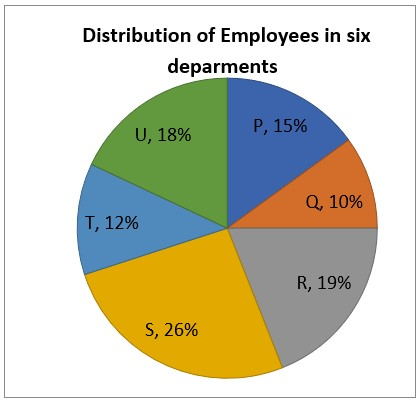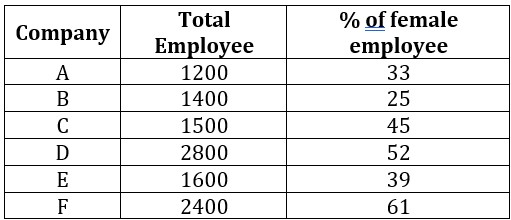1. If 25% of employees working in ‘P’ department of company A are females, then find the ratio b/w males working in ‘P’ department of company A and number of employees working in’T’ department of company E?

(a) 22 : 31

(b) 45 : 64

(c) 47 : 61

(d) 37 : 42

(e) 42 : 611. If each male and female employee of company D get Rs 280 and Rs 250 per day respectively and 25% females of company D work in R department, then find the total salary distributed in R department of same company ?

(a) Rs 125040

(b) Rs 160400

(c) Rs 150400

(d) Rs 138040

(e) Rs 105040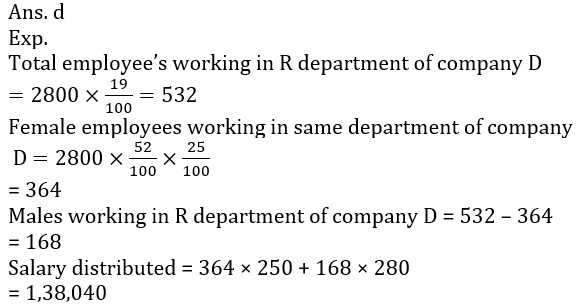1. The average of employees working in P, Q, R department of company C are approximate what percent more/less than average of employees working in S and T department of company F?

(a) 68%

(b) 37%

(c) 52%

(d) 49%

(e) 28%1. If 25% employees of company B resigns and joins company D, then find the difference between employees working in ‘U’ department of both companies? (consider % distribution of employees in different departments remains same).

(a) 378

(b) 400

(c) 512

(d) 394

(e) 420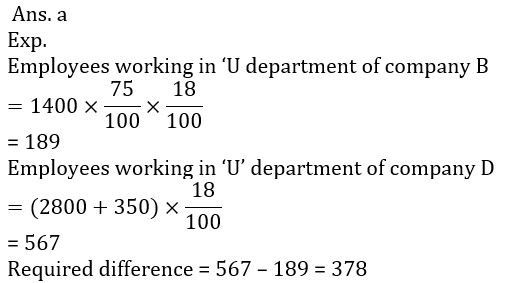Directions (5-8): The following questions are accompanied by two statements (i), (ii) and (iii). You have to determine which statement(s) is/are necessary/sufficient to answer the question.

1. Three friend P, Q and R earn a profit of 2100 Rs. Find the profit of Q.

(I) Share of R in profit is 40% of what Q and P got together.

(II) Share of P 100/3% more than the share of R.

(III) Share of P and R is 100% more than the share of Q in total profit.

(a) (I) and (II) together

(b) Only (III) or (I) and (II) together

(c) Only (I) or Only (III)

(d) All statements required.

(e) Answer cannot be found even using all statements.1. A bag contains three types of balls, Red, Blue, Black. Find the number of balls of black color.

(I) Number of blue balls are 40% of the total balls.

(II) Probability of getting one red ball and one blue ball are ¼ and ⅖ respectively.

(III) If one green ball adds in the bag then probability of getting black ball become ⅓.

(a) Only (I)

(b) Both (I) and (II) together

(c) Only (I) or Only (II)

(d) Both (II) and (III) together

(e) Answer cannot be found even using all statements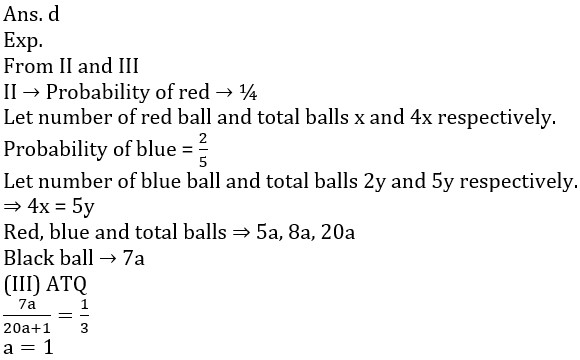1. What is the sum of present age of K and L.

(I) Four year ago ratio of age of K and L is 7 : 5.

(II) Sum of present age of L and N together is equal to the sum of present age of K and M.

(III) M is eight years younger than N.

(a) (I) and (II) together

(b) Only (III) or (I) and (II) together

(c) Only (i) or Only (iii)

(d) All statements required.

(e) answer cannot be found even using all statements1. Find the distance between Rampur and Kanpur.

(I) A car takes 8 hours to go Rampur from Kanpur.

(II) If car start from Rampur and a motorbike from Kanpur they meet after 5 hours.

(III) Difference between speed of car and motorbike is 20 km/hr.

(a) (I) and (II) together

(b) Only (III) or (I) and (II) together

(c) Only (i) or Only (iii)

(d) All statements required.

(e) answer cannot be found even using all statements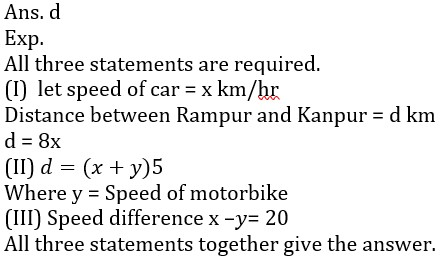1. A shopkeeper gives a discount of 12% on marked price of article P and cost price of another article Q is 100/11% more than selling price of article P. If shopkeeper sold article Q at 50/3 % profit and selling price of article Q was Rs. 720 more than selling price of article P, then find the cost price of article Q ?

(a) 3880 Rs.

(b) 4480 Rs.

(c) 5250 Rs.

(d) 2880 Rs.

(e) 3580 Rs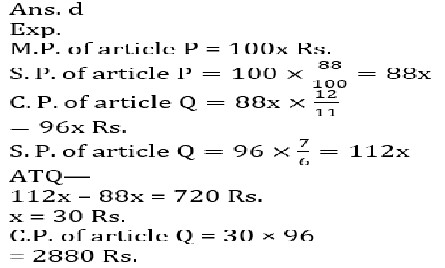1. Ratio between speed of a truck and a bus is 17 : 18 and also a train covered 540 km in 21600 seconds. If speed of bus is 4/5 th of speed of train then find distance covered by truck in 18000 seconds ?

(a) 280km

(b) 340km

(c) 270km

(d) 320km

(e) 360 km